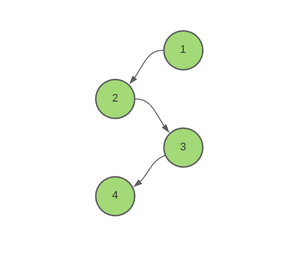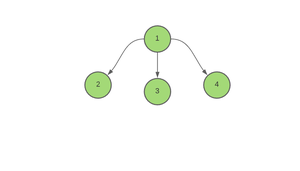Related Articles

# Minimum time required to color all edges of a Tree

• Difficulty Level : Hard
• Last Updated : 08 Sep, 2021

Given an array of pairs Edges[][], representing edges connecting vertices in a Tree consisting of N nodes, the task is to find the minimum time required to color all the edges of a Tree based on the assumption that coloring an edge requires 1 unit of time.

Note: Multiple edges can be colored on a particular instant, but a node can be part of only one of the edges colored on a particular day.

Examples

Input: Edges[][] = ((1, 2), (3, 4), (2, 3))Output: 2
Explanation:
Step 1: Color edges (1, 2) and (3, 4)
Step 2: Color edge (2, 3)

Input: Edges[][] = ((1, 2), (1, 3), (1, 4))Output : 3

Approach: This problem can be solved using DFS(Depth First Search). Follow the steps below to solve the problem:

• Initialize global variables, say ans as 0, to store the minimum time required to color all the edges of a Tree.
• Initialize a variable current_time as 0, to store the time required to color the current edge.
• Iterate over the children of the current node and perform the following steps:
• If the current edge is not visited, i.e the current node is not equal to the parent node:
• Increase current_time by 1.
• Check if the parent edge has been colored at the same time or not. If found to be true, then increase current_time by 1 as a node cannot be part of more than one edge which are being colored at the same time.
• Update ans as maximum of ans and current_time.
• Call the recursive function minTimeToColor for the children of the current node.
• After the end of this function, print ans.

Below is the code for the above approach.

## C++

 `// C++ program for the above approach` `#include ``using` `namespace` `std;` `// Stores the required answer``int` `ans = 0;` `// Stores the graph``vector<``int``> edges;` `// Function to add edges``void` `Add_edge(``int` `u, ``int` `v)``{``    ``edges[u].push_back(v);``    ``edges[v].push_back(u);``}` `// Function to calculate the minimum time``// required to color all the edges of a tre``void` `minTimeToColor(``int` `node, ``int` `parent,``                    ``int` `arrival_time)``{``    ``// Starting from time = 0,``    ``// for all the child edges``    ``int` `current_time = 0;` `    ``for` `(``auto` `x : edges[node]) {` `        ``// If the edge is not visited yet.``        ``if` `(x != parent) {` `            ``// Time of coloring of``            ``// the current edge``            ``++current_time;` `            ``// If the parent edge has``            ``// been colored at the same time``            ``if` `(current_time == arrival_time)``                ``++current_time;` `            ``// Update the maximum time``            ``ans = max(ans, current_time);` `            ``// Recursively call the``            ``// function to its child node``            ``minTimeToColor(x, node, current_time);``        ``}``    ``}``}` `// Driver Code``int` `main()``{` `    ``pair<``int``, ``int``> A[] = { { 1, 2 },``                           ``{ 2, 3 },``                           ``{ 3, 4 } };` `    ``for` `(``auto` `i : A) {` `        ``Add_edge(i.first, i.second);``    ``}` `    ``// Function call``    ``minTimeToColor(1, -1, 0);` `    ``// Finally, print the answer``    ``cout << ans << ``"\n"``;``}`

## Java

 `// Java program for the above approach``import` `java.util.*;` `class` `GFG{` `// Stores the required answer``static` `int` `ans = ``0``;` `// Stores the graph``@SuppressWarnings``(``"unchecked"``)``static` `Vector edges[] = ``new` `Vector[``100000``];` `// Function to add edges``static` `void` `Add_edge(``int` `u, ``int` `v)``{``    ``edges[u].add(v);``    ``edges[v].add(u);``}` `// Function to calculate the minimum time``// required to color all the edges of a tre``static` `void` `minTimeToColor(``int` `node, ``int` `parent,``                           ``int` `arrival_time)``{``    ` `    ``// Starting from time = 0,``    ``// for all the child edges``    ``int` `current_time = ``0``;` `    ``for``(``int` `x = ``0``; x < edges[node].size(); x++)``    ``{``        ` `        ``// If the edge is not visited yet.``        ``if` `(edges[node].get(x) != parent)``        ``{``            ` `            ``// Time of coloring of``            ``// the current edge``            ``++current_time;` `            ``// If the parent edge has``            ``// been colored at the same time``            ``if` `(current_time == arrival_time)``                ``++current_time;` `            ``// Update the maximum time``            ``ans = Math.max(ans, current_time);` `            ``// Recursively call the``            ``// function to its child node``            ``minTimeToColor(edges[node].get(x), node,``                           ``current_time);``        ``}``    ``}``}` `// Driver Code``public` `static` `void` `main(String[] args)``{``    ``for``(``int` `i = ``0``; i < edges.length; i++)``        ``edges[i] = ``new` `Vector();``        ` `    ``int` `A[][] = { { ``1``, ``2` `},``                  ``{ ``2``, ``3` `},``                  ``{ ``3``, ``4` `} };` `    ``for``(``int` `i = ``0``; i < ``3``; i++)``    ``{``        ``Add_edge(A[i][``0``], A[i][``1``]);``    ``}``    ` `    ``// Function call``    ``minTimeToColor(``1``, -``1``, ``0``);` `    ``// Finally, print the answer``    ``System.out.print(ans + ``"\n"``);``}``}` `// This code is contributed by umadevi9616`

## Python3

 `# Python3 program for the above approach` `# Stores the required answer``ans ``=` `0` `# Stores the graph``edges ``=` `[[] ``for` `i ``in` `range``(``100000``)]` `# Function to add edges``def` `Add_edge(u, v):``    ` `    ``global` `edges``    ``edges[u].append(v)``    ``edges[v].append(u)` `# Function to calculate the minimum time``# required to color all the edges of a tre``def` `minTimeToColor(node, parent, arrival_time):``    ` `    ``global` `ans``    ` `    ``# Starting from time = 0,``    ``# for all the child edges``    ``current_time ``=` `0` `    ``for` `x ``in` `edges[node]:` `        ``# If the edge is not visited yet.``        ``if` `(x !``=` `parent):` `            ``# Time of coloring of``            ``# the current edge``            ``current_time ``+``=` `1` `            ``# If the parent edge has``            ``# been colored at the same time``            ``if` `(current_time ``=``=` `arrival_time):``                ``current_time ``+``=` `1` `            ``# Update the maximum time``            ``ans ``=` `max``(ans, current_time)` `            ``# Recursively call the``            ``# function to its child node``            ``minTimeToColor(x, node, current_time)` `# Driver Code``if` `__name__ ``=``=` `'__main__'``:``    ` `    ``A ``=` `[ [ ``1``, ``2` `],``          ``[ ``2``, ``3` `],``          ``[ ``3``, ``4` `] ]` `    ``for` `i ``in` `A:``        ``Add_edge(i[``0``], i[``1``])` `    ``# Function call``    ``minTimeToColor(``1``, ``-``1``, ``0``)` `    ``# Finally, print the answer``    ``print``(ans)` `# This code is contributed by mohit kumar 29`

## C#

 `// C# program for the above approach``using` `System;``using` `System.Collections.Generic;``class` `GFG {``    ` `    ``// Stores the required answer``    ``static` `int` `ans = 0;``     ` `    ``// Stores the graph``    ``static` `List> edges = ``new` `List>();``     ` `    ``// Function to add edges``    ``static` `void` `Add_edge(``int` `u, ``int` `v)``    ``{``        ``edges[u].Add(v);``        ``edges[v].Add(u);``    ``}``    ` `    ``// Function to calculate the minimum time``    ``// required to color all the edges of a tre``    ``static` `void` `minTimeToColor(``int` `node, ``int` `parent,``                        ``int` `arrival_time)``    ``{``        ``// Starting from time = 0,``        ``// for all the child edges``        ``int` `current_time = 0;``     ` `        ``for``(``int` `x = 0; x < edges[node].Count; x++) {``     ` `            ``// If the edge is not visited yet.``            ``if` `(edges[node][x] != parent) {``     ` `                ``// Time of coloring of``                ``// the current edge``                ``++current_time;``     ` `                ``// If the parent edge has``                ``// been colored at the same time``                ``if` `(current_time == arrival_time)``                    ``++current_time;``     ` `                ``// Update the maximum time``                ``ans = Math.Max(ans, current_time);``     ` `                ``// Recursively call the``                ``// function to its child node``                ``minTimeToColor(edges[node][x], node, current_time);``            ``}``        ``}``    ``}` `  ``// Driver code``  ``static` `void` `Main() {``    ` `    ``for``(``int` `i = 0; i < 100000; i++)``    ``{``        ``edges.Add(``new` `List<``int``>());``    ``}``    ` `    ``int``[,] A = { { 1, 2 }, { 2, 3 }, { 3, 4 } };`` ` `    ``for``(``int` `i = 0; i < 3; i++)``    ``{``        ``Add_edge(A[i,0], A[i,1]);``    ``}`` ` `    ``// Function call``    ``minTimeToColor(1, -1, 0);`` ` `    ``// Finally, print the answer``    ``Console.WriteLine(ans);``  ``}``}` `// This code is contributed by divyeshrabadiya07.`

## Javascript

 ``
Output:
`2`

Time Complexity: O(N)
Auxiliary Spacey: O(N)

Attention reader! Don’t stop learning now. Get hold of all the important DSA concepts with the DSA Self Paced Course at a student-friendly price and become industry ready.  To complete your preparation from learning a language to DS Algo and many more,  please refer Complete Interview Preparation Course.

In case you wish to attend live classes with experts, please refer DSA Live Classes for Working Professionals and Competitive Programming Live for Students.

My Personal Notes arrow_drop_up